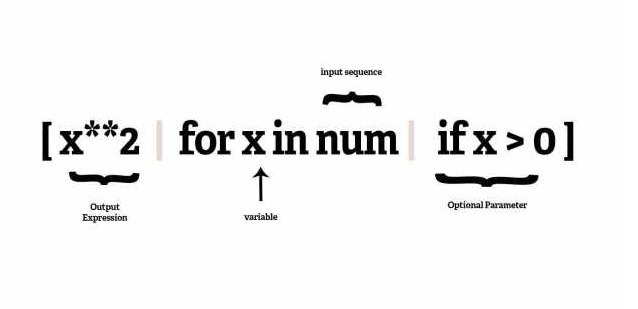# Python高级编程技巧

• 一个输入序列

• 一个表示输入序列成员的变量

• 一个可选的断言表达式

• 一个将输入序列中满足断言表达式的成员变换成输出列表成员的输出表达式

num = [1, 4, -5, 10, -7, 2, 3, -1]

filtered_and_squared = []

for number in num:

if number > 0:

filtered_and_squared.append(number ** 2)

print filtered_and_squared

# [1, 16, 100, 4, 9]

num = [1, 4, -5, 10, -7, 2, 3, -1]

filtered_and_squared = map(lambda x: x ** 2, filter(lambda x: x > 0, num))

print filtered_and_squared

# [1, 16, 100, 4, 9]

num = [1, 4, -5, 10, -7, 2, 3, -1]

filtered_and_squared = [ x**2 for x in num if x > 0]

print filtered_and_squared

# [1, 16, 100, 4, 9]• 迭代器(iterator)遍历输入序列num的每个成员x

• 断言式判断每个成员是否大于零

• 如果成员大于零，则被交给输出表达式，平方之后成为输出列表的成员。

num = [1, 4, -5, 10, -7, 2, 3, -1]

filtered_and_squared = ( x**2 for x in num if x > 0 )

print filtered_and_squared

# <generator object <genexpr> at 0x00583E18>

for item in filtered_and_squared:

print item

# 1, 16, 100 4,9

num = [1, 4, -5, 10, -7, 2, 3, -1]

def square_generator(optional_parameter):

return (x ** 2 for x in num if x > optional_parameter)

print square_generator(0)

# <generator object <genexpr> at 0x004E6418>

# Option I

for k in square_generator(0):

print k

# 1, 16, 100, 4, 9

# Option II

g = list(square_generator(0))

print g

# [1, 16, 100, 4, 9]

alist = ['a1', 'a2', 'a3']

blist = ['1', '2', '3']

for a, b in zip(alist, blist):

print a, b

# a1 1

# a2 2

# a3 3

import os

def tree(top):

for path, names, fnames in os.walk(top):

for fname in fnames:

yield os.path.join(path, fname)

print name

def timethis(func):

'''

Decorator that reports the execution time.

'''

pass

@timethis

def countdown(n):

while n > 0:

n -= 1

import time

from functools import wraps

def timethis(func):

'''

Decorator that reports the execution time.

'''

@wraps(func)

def wrapper(*args, **kwargs):

start = time.time()

result = func(*args, **kwargs)

end = time.time()

print(func.__name__, end-start)

return result

return wrapper

@timethis

def countdown(n):

while n > 0:

n -= 1

countdown(100000)

# ('countdown', 0.006999969482421875)

@timethis

def countdown(n):

def countdown(n):

...

countdown = timethis(countdown)

class decorator(object):

def __init__(self, f):

print("inside decorator.__init__()")

f() # Prove that function definition has completed

def __call__(self):

print("inside decorator.__call__()")

@decorator

def function():

print("inside function()")

print("Finished decorating function()")

function()

# inside decorator.__init__()

# inside function()

# Finished decorating function()

# inside decorator.__call__()

1. 语法糖@decorator相当于function=decorator(function)，在此调用decorator的__init__打印“inside decorator.__init__()”

2. 随后执行f()打印“inside function()”

3. 随后执行“print(“Finished decorating function()”)”

4. 最后在调用function函数时，由于使用装饰器包装，因此执行decorator的__call__打印 “inside decorator.__call__()”。

def decorator(func):

def modify(*args, **kwargs):

variable = kwargs.pop('variable', None)

print variable

x,y=func(*args, **kwargs)

return x,y

return modify

@decorator

def func(a,b):

print a**2,b**2

return a**2,b**2

func(a=4, b=5, variable="hi")

func(a=4, b=5)

# hi

# 16 25

# None

# 16 25

contextlib模块包含了与上下文管理器和with声明相关的工具。通常如果你想写一个上下文管理器，则你需要定义一个类包含__enter__方法以及__exit__方法，例如：

import time

class demo:

def __init__(self, label):

self.label = label

def __enter__(self):

self.start = time.time()

def __exit__(self, exc_ty, exc_val, exc_tb):

end = time.time()

print('{}: {}'.format(self.label, end - self.start))

import time

class demo:

def __init__(self, label):

self.label = label

def __enter__(self):

self.start = time.time()

def __exit__(self, exc_ty, exc_val, exc_tb):

end = time.time()

print('{}: {}'.format(self.label, end - self.start))

with demo('counting'):

n = 10000000

while n > 0:

n -= 1

# counting: 1.36000013351

1. __enter__方法，当执行流进入with代码块时，__enter__方法将执行。并且它将返回一个可供上下文使用的对象。

2. 当执行流离开with代码块时，__exit__方法被调用，它将清理被使用的资源。

from contextlib import contextmanager

import time

@contextmanager

def demo(label):

start = time.time()

try:

yield

finally:

end = time.time()

print('{}: {}'.format(label, end - start))

with demo('counting'):

n = 10000000

while n > 0:

n -= 1

# counting: 1.32399988174

• __get__(self, instance, owner) – 这个方法是当属性被通过(value = obj.attr)的方式获取时调用，这个方法的返回值将被赋给请求此属性值的代码部分。

• __set__(self, instance, value) – 这个方法是当希望设置属性的值(obj.attr = ‘value’)时被调用，该方法不会返回任何值。

• __delete__(self, instance) – 当从一个对象中删除一个属性时(del obj.attr)，调用此方法。

class Celsius(object):

def __init__(self, value=0.0):

self.value = float(value)

def __get__(self, instance, owner):

return self.value

def __set__(self, instance, value):

self.value = float(value)

class Temperature(object):

celsius = Celsius()

temp=Temperature()

temp.celsius #calls Celsius.__get__

import weakref

class lazyattribute(object):

def __init__(self, f):

self.data = weakref.WeakKeyDictionary()

self.f = f

def __get__(self, obj, cls):

if obj not in self.data:

self.data[obj] = self.f(obj)

return self.data[obj]

class Foo(object):

@lazyattribute

def bar(self):

print "Being lazy"

return 42

f = Foo()

print f.bar

# Being lazy

# 42

print f.bar

# 42

1. 柯里化

2. function，method，bound method及unbound method的区别。首先，函数(function)是由def或lambda创建的。当一个函数在class语句块中定义或是由type来创建时，它会转成一个非绑定方法(unbound method)，而当通过类实例(instance)来访问此方法的时候，它将转成绑定方法(bound method)，绑定方法会自动将实例作为第一个参数传入方法。综上所述，方法是出现在类中的函数，绑定方法是一个绑定了具体实例的方法，反之则是非绑定方法。

class demo(object):

pass

obj = demo()

print "Class of obj is {0}".format(obj.__class__)

print "Class of obj is {0}".format(demo.__class__)

# Class of obj is <class '__main__.demo'>

# Class of obj is <type 'type'>

• 当看见一个类定义，python会收集所有属性到一个字典中。

• 当类定义结束，python将决定类的元类，我们就称它为Meta吧。

• 最后，python执行Meta(name, bases, dct)，其中：

a. Meta是元类，所以这个调用是实例化它。

b. name是新建类的类名。

c. bases是新建类的基类元组

d. dct将属性名映射到对象，列出所有的类属性。

“请求宽恕比请求许可更容易(EFAP)”

Null对象

Null对象能够用来代替None类型以避免对None的测试。